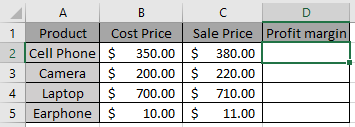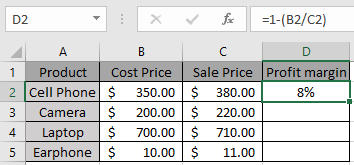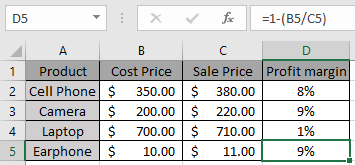# How to Calculate Profit margin percentage in Excel

In this article, we will learn about how to Calculate Profit margin percentage of the set of data provided in Excel.

Profit margin percentage calculates the percentage change in profit margin using a simple mathematical operation shown below in the box.
Mathematical formula:

Profit margin percentage = 1 - (cost price / sale price)

We will use this in Excel to find Percentage change in profit margin.
Let’s use the above formula on a few examples to learn betterUse the Formula in D2 cell

=1-(B2/C2)As you can see in the above snapshot first data percentage of profit margin is 8%.

Copy the formula in the remaining cells to get the percentage change of profit margin for the rest of the data.Profit margin percent can be calculated using the above method.

Hope you understood how to calculate the Percentage margin profit of a set of values. Explore more articles on Mathematical formulation in Excel here. Mention your queries in the comment box below. We will help you with it.

Related Articles:

How to use the IPMT Function in Excel

How to use the PMT Function in Excel

How to use the XNPV Function in Excel

How to use the PRICE Function in Excel

How to use the CUMIPMT Function in Excel

How to use the RATE Function in Excel

Popular Articles

50 Excel Shortcut to Increase Your Productivity : Get faster at your task. These 50 shortcuts will make you work even faster on Excel.

How to use the VLOOKUP Function in Excel : This is one of the most used and popular functions of excel that is used to lookup value from different ranges and sheets.

How to use the COUNTIF function in Excel : Count values with conditions using this amazing function. You don't need to filter your data to count specific values. Countif function is essential to prepare your dashboard.

How to use the SUMIF Function in Excel : This is another dashboard essential function. This helps you sum up values on specific conditions.

Terms and Conditions of use

The applications/code on this site are distributed as is and without warranties or liability. In no event shall the owner of the copyrights, or the authors of the applications/code be liable for any loss of profit, any problems or any damage resulting from the use or evaluation of the applications/code.